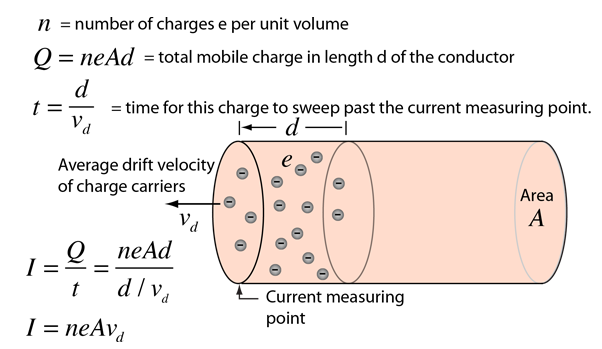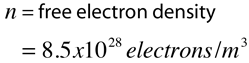# Microscopic Electric Current

Since electric charge is quantized in discrete multiples of the electron charge, it is instructive to look at electric current as the movement of multiple microscopic charge carriers with a drift velocity in a conductor.Microscopic view of Ohm's law
Index

Electric current

 HyperPhysics***** Electricity and Magnetism R Nave
Go Back

# Electric Charge Carriers

For electric current in a copper wire, the charge carriers are the mobile electrons and the positively charged copper ions are essentially stationary in the metal lattice. Nevertheless, treatments of electric circuits usually use conventional current, as if positive charges were moving. Debate continues about this practice, but the physical nature of the charge carriers in copper is fairly straightforward.

In other applications of electric current however, the identification of the charge carriers is not so simple. In semiconductors, for example, you sometimes have electrons which are mobile, and sometimes have deficiencies of electrons, called "holes" which are mobile. There are significant differences in the way they conduct. One way to detect which kind of conduction is taking place is with the Hall effect, which gives a different polarity for the Hall voltage for positive and negative charge carriers. In many substances, electric conduction is not just free electron movement.

 Charge carriers in Hall effect
Index

Electric current

 HyperPhysics***** Electricity and Magnetism R Nave
Go Back

# Drift Velocity of Charge Carriers

Although your light turns on very quickly when you flip the switch, and you find it impossible to flip off the light and get in bed before the room goes dark, the actual drift velocity of electrons through copper wires is very slow. It is the change or "signal" which propagates along wires at essentially the speed of light.

The drift velocity of electrons in a copper wire can be calculated fromShowShow

If the wire diameter is mm then the area is A = x10^ m2.
For current I = amperes,
 the drift velocity is Vd = x10^ m/s = cm/hour.

This slow average drift speed for electrons is tiny compared to the average electron speed associated with its internal energy.

Calculation note: Any of the properties of the wire can be changed. Parameters left unspecified default to values for a 12 gauge copper wire carrying 10 amperes.

Index

Electric current

 HyperPhysics***** Electricity and Magnetism R Nave
Go Back

# Density of Charge Carriers

Calculation of the density of free electrons in a metal like copper involves the basic physical data about the metal, plus the fact that copper provides about one free electron per atom to the electrical conduction process. A representative value can be calculated with the following data.Relate to microscopic view of Ohm's law
Index

Electric current

 HyperPhysics***** Electricity and Magnetism R Nave
Go Back